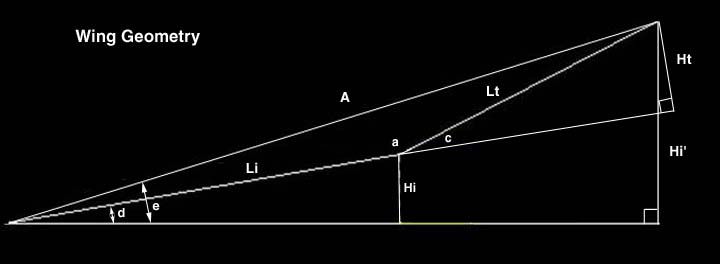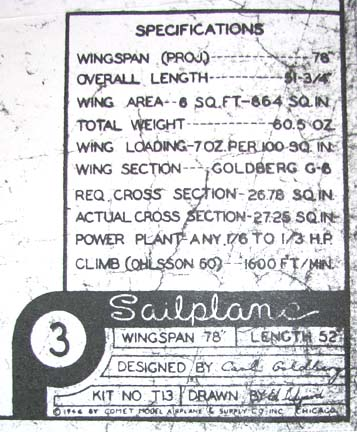Sailplane Construction Project Session #104

Comet Sailplane Project

The Comet Sailplane plan shows the rise in the wing's tip panel to be 4-3/8" with the wing's inner panel flat on the work table. Unfortunately, to get the proper elevation in the wing's inner panel, the plan shows a 10.0" rise out at the tip panel, which is awkward to get jigged up. Therefore, I wanted to determine (calculate) the rise of inner panel at the polyhedral joint, which is much easier to set up from a jig standpoint. When I first went through the geometry to determine the rise of inner panel at the polyhedral joint, I made a number of errors in my calculations %\$#@& . By consulting with Sergio Montes, Jim O'Reilly, and Alfredo Herbon, they were able to quickly straighten me out. The correct rise  of inner panel at the polyhedral joint is 3.3736". For documentation purposes, I want to present the calculation approach, which is shown below.

Wing Geometry Modified from Alfredo Herbon's CAD DrawingGiven:
Sc = 12.00" (Center Panel Span)
Lt = 14.40" (Tip Panel Span)
Ht = 4-3/8" (4.3750") (Tip Panel Span Elevation)
Li = 20.00" (Inner Panel Span)
Hi' = 10.00" (Inner Panel Elevation at Tip Panel)
Calculations:
c = ArcSin(Ht/Lt) = 17.6872 Deg (Polyhedral Angle)
A*A = (Lt*Lt + Li*Li - 2*Lt*Li *Cos a)
a = 180-c
Cos a = Cos(180-c) = - Cos c
A*A = (Lt*Lt + Li*Li + 2*Lt*Li *Cos c)
A = 34.0019"
b = ArcSin(Ht/A) = 7.3927 Deg
e = ArcSin(Hi'/A) = 17.1036Deg
d = e-b = 9.7109 Deg (Dihedral Angle)

Hi = Li*Sin(d) = 3.3736" (Rise  of inner panel at the polyhedral joint)

With this information, the flat plan wing span is calculated as [2*(14.4 + 20.0) + 12.0] = 80.80", and the projected wing span is calculated as [2*(A*Cos e) + 12.0] = 77.00". Interesting enough, neither one of these values agree with the 78" wing span quoted in the specification title block on the Comet plans shown below..................Tandy